# Law of inertia

12 de Jul de 2016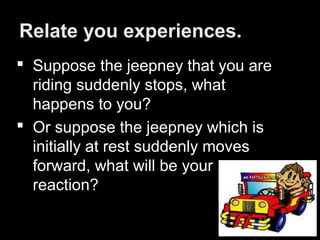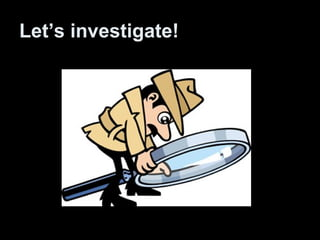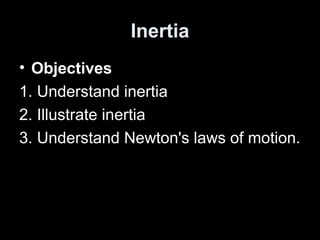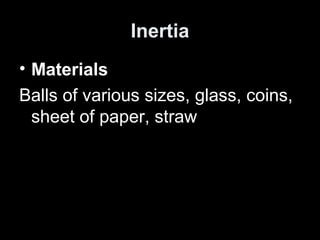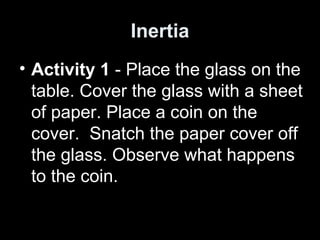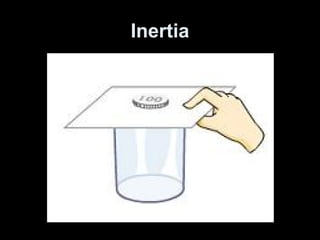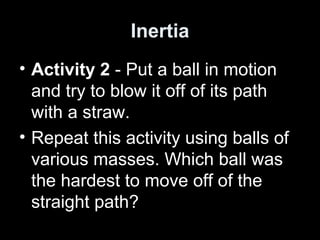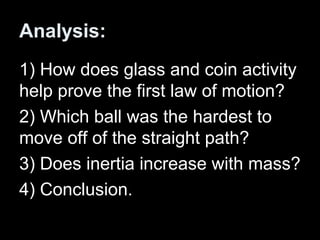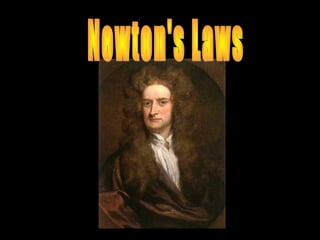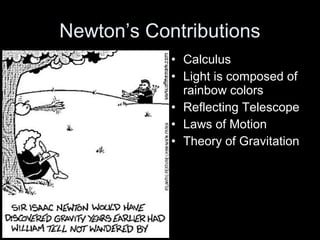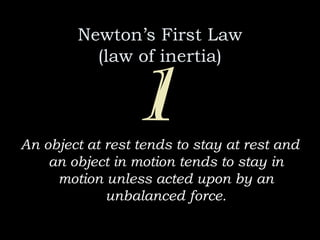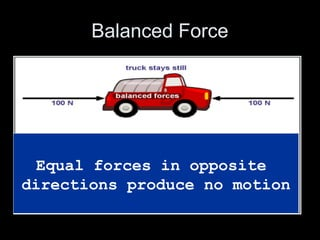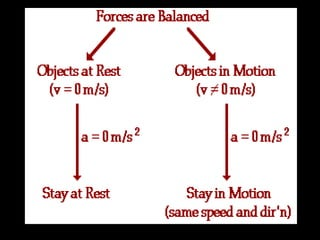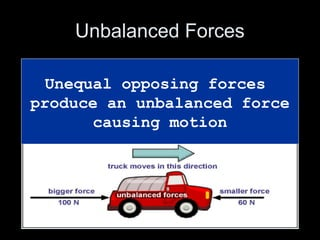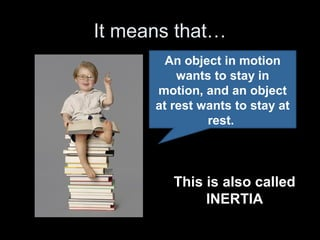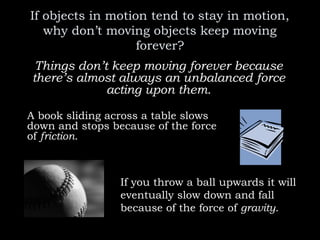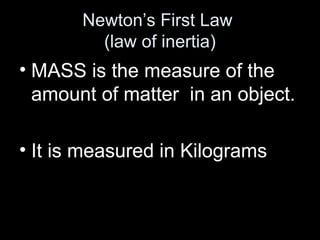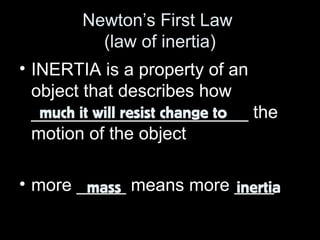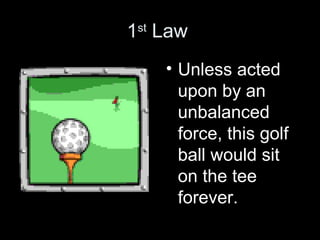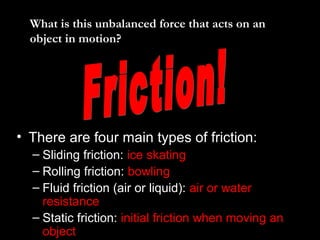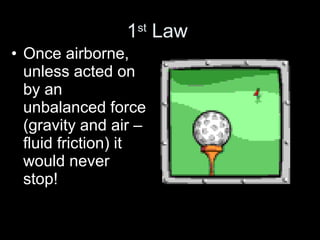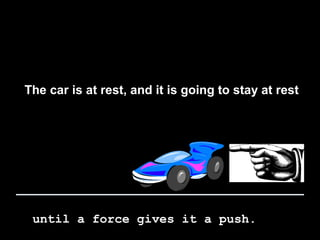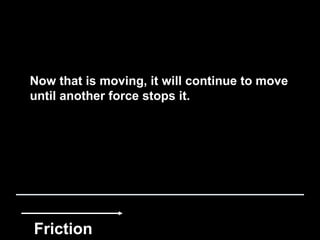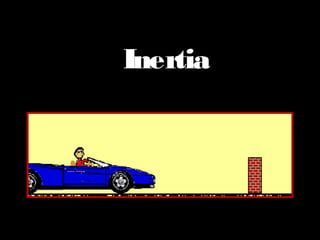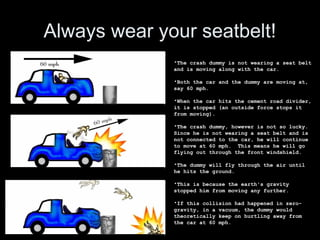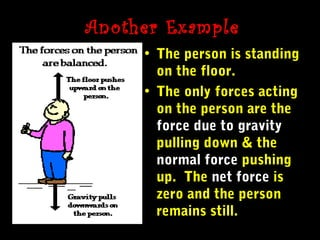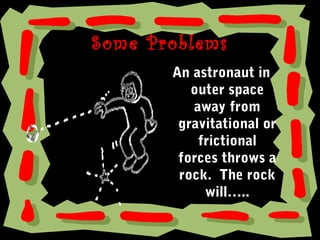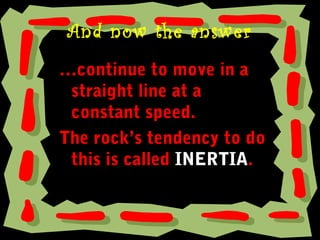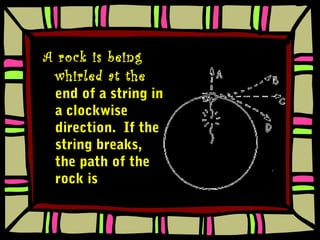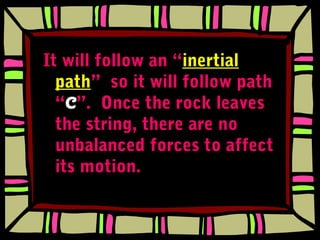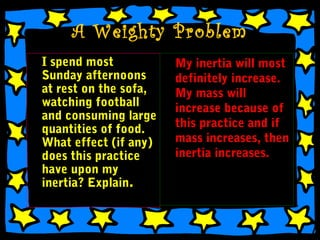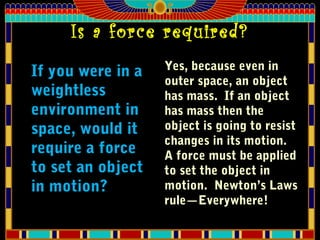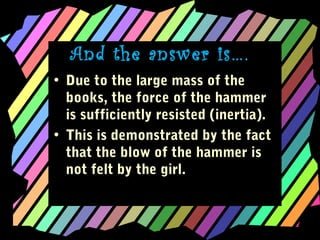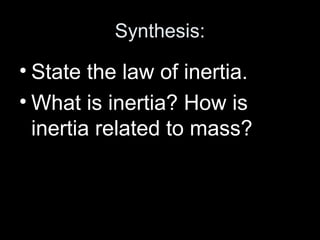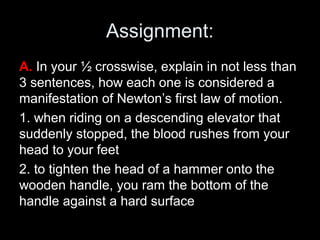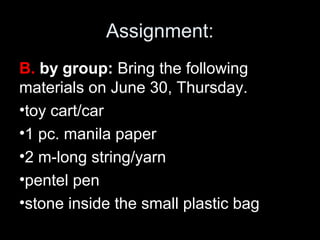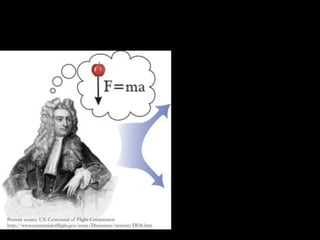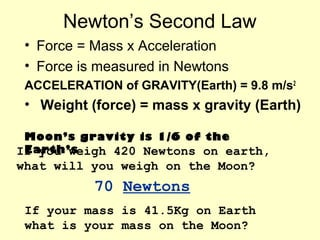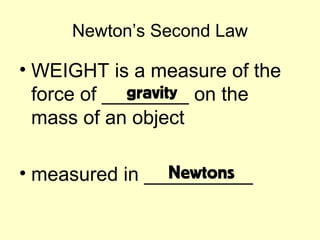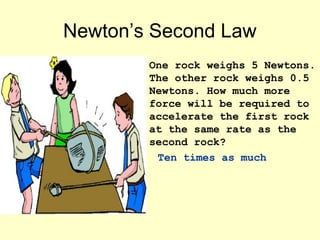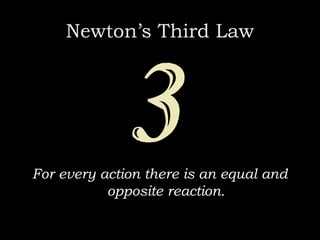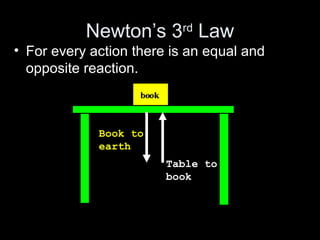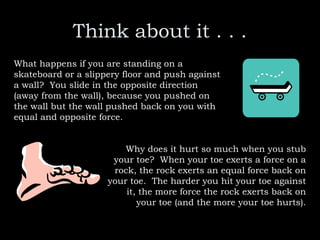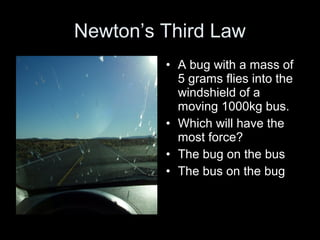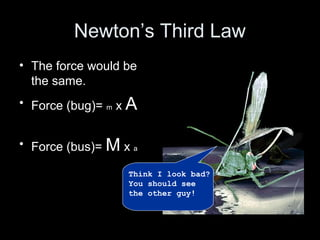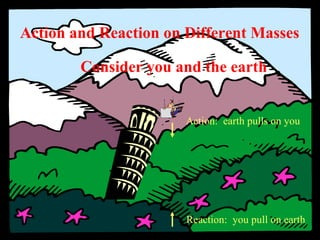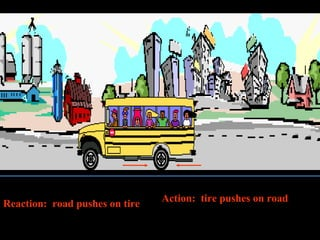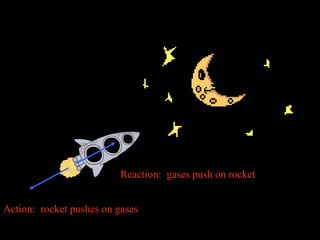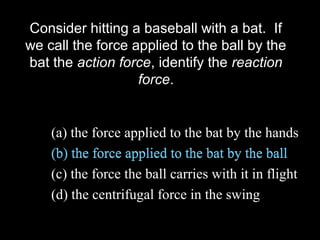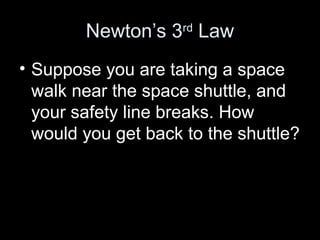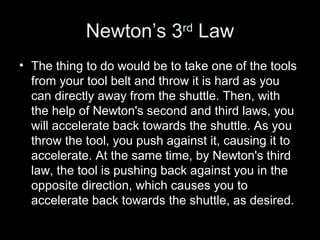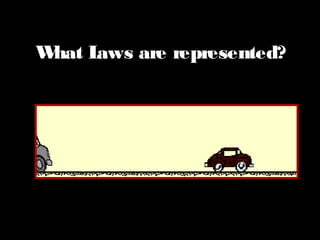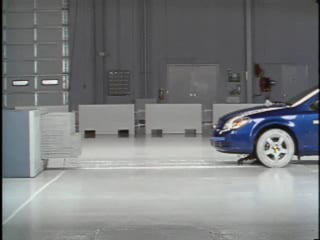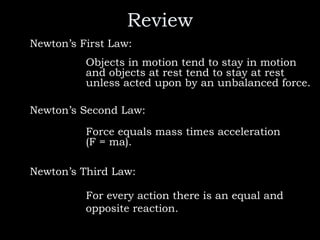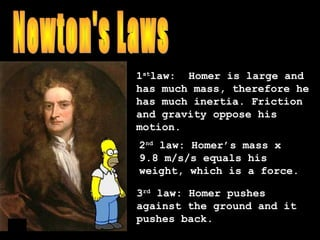1 de 58

### Law of inertia

• 2. Relate you experiences.  Suppose the jeepney that you are riding suddenly stops, what happens to you?  Or suppose the jeepney which is initially at rest suddenly moves forward, what will be your reaction?
• 4. Inertia • Objectives 1. Understand inertia 2. Illustrate inertia 3. Understand Newton's laws of motion.
• 5. Inertia • Materials Balls of various sizes, glass, coins, sheet of paper, straw
• 6. Inertia • Activity 1 - Place the glass on the table. Cover the glass with a sheet of paper. Place a coin on the cover. Snatch the paper cover off the glass. Observe what happens to the coin.
• 8. Inertia • Activity 2 - Put a ball in motion and try to blow it off of its path with a straw. • Repeat this activity using balls of various masses. Which ball was the hardest to move off of the straight path?
• 9. Analysis: 1) How does glass and coin activity help prove the first law of motion? 2) Which ball was the hardest to move off of the straight path? 3) Does inertia increase with mass? 4) Conclusion.
• 11. Newton’s Contributions • Calculus • Light is composed of rainbow colors • Reflecting Telescope • Laws of Motion • Theory of Gravitation
• 12. Newton’s First Law (law of inertia) An object at rest tends to stay at rest and an object in motion tends to stay in motion unless acted upon by an unbalanced force.
• 13. Balanced Force Equal forces in opposite directions produce no motion
• 15. Unbalanced Forces Unequal opposing forces produce an unbalanced force causing motion
• 16. It means that… An object in motion wants to stay in motion, and an object at rest wants to stay at rest. This is also called INERTIA
• 17. If objects in motion tend to stay in motion, why don’t moving objects keep moving forever? Things don’t keep moving forever because there’s almost always an unbalanced force acting upon them. A book sliding across a table slows down and stops because of the force of friction. If you throw a ball upwards it will eventually slow down and fall because of the force of gravity.
• 18. Newton’s First Law (law of inertia) • MASS is the measure of the amount of matter in an object. • It is measured in Kilograms
• 19. Newton’s First Law (law of inertia) • INERTIA is a property of an object that describes how ______________________ the motion of the object • more _____ means more ____ much it will resist change to mass inertia
• 20. 1st Law • Unless acted upon by an unbalanced force, this golf ball would sit on the tee forever.
• 21. • There are four main types of friction: – Sliding friction: ice skating – Rolling friction: bowling – Fluid friction (air or liquid): air or water resistance – Static friction: initial friction when moving an object What is this unbalanced force that acts on anWhat is this unbalanced force that acts on an object in motion?object in motion?
• 22. 1st Law • Once airborne, unless acted on by an unbalanced force (gravity and air – fluid friction) it would never stop!
• 23. The car is at rest, and it is going to stay at rest until a force gives it a push.
• 24. Now that is moving, it will continue to move until another force stops it. Friction
• 25. The best place to test inertia is in Space, why? In outer space there is no air, or anything else to create friction. So moving objects will keep moving forever, and objects that aren't moving will stand still forever.
• 27. Always wear your seatbelt! •The crash dummy is not wearing a seat belt and is moving along with the car. •Both the car and the dummy are moving at, say 60 mph. •When the car hits the cement road divider, it is stopped (an outside force stops it from moving). •The crash dummy, however is not so lucky. Since he is not wearing a seat belt and is not connected to the car, he will continue to move at 60 mph. This means he will go flying out through the front windshield. •The dummy will fly through the air until he hits the ground. •This is because the earth's gravity stopped him from moving any further. •If this collision had happened in zero- gravity, in a vacuum, the dummy would theoretically keep on hurtling away from the car at 60 mph.
• 28. Another Example • The person is standing on the floor. • The only forces acting on the person are the force due to gravity pulling down & the normal force pushing up. The net force is zero and the person remains still.
• 29. Some Problems An astronaut in outer space away from gravitational or frictional forces throws a rock. The rock will…..
• 30. And now the answer …continue to move in a straight line at a constant speed. The rock’s tendency to do this is called INERTIA.
• 31. A rock is being whirled at the end of a string in a clockwise direction. If the string breaks, the path of the rock is
• 32. It will follow an “inertial path” so it will follow path “C”. Once the rock leaves the string, there are no unbalanced forces to affect its motion.
• 33. A Weighty Problem I spend most Sunday afternoons at rest on the sofa, watching football and consuming large quantities of food. What effect (if any) does this practice have upon my inertia? Explain. My inertia will most definitely increase. My mass will increase because of this practice and if mass increases, then inertia increases.
• 34. Is a force required? If you were in a weightless environment in space, would it require a force to set an object in motion? Yes, because even in outer space, an object has mass. If an object has mass then the object is going to resist changes in its motion. A force must be applied to set the object in motion. Newton’s Laws rule—Everywhere!
• 35. A Final Problem Why isn't the girl hurt when the nail is driven into the block of wood?
• 36. And the answer is…. • Due to the large mass of the books, the force of the hammer is sufficiently resisted (inertia). • This is demonstrated by the fact that the blow of the hammer is not felt by the girl.
• 37. Synthesis: • State the law of inertia. • What is inertia? How is inertia related to mass?
• 38. Assignment: A. In your ½ crosswise, explain in not less than 3 sentences, how each one is considered a manifestation of Newton’s first law of motion. 1. when riding on a descending elevator that suddenly stopped, the blood rushes from your head to your feet 2. to tighten the head of a hammer onto the wooden handle, you ram the bottom of the handle against a hard surface
• 39. Assignment: B. by group: Bring the following materials on June 30, Thursday. •toy cart/car •1 pc. manila paper •2 m-long string/yarn •pentel pen •stone inside the small plastic bag
• 40. Newton’s Second Law Force equals mass times acceleration. F = ma
• 41. Newton’s Second Law • Force = Mass x Acceleration • Force is measured in Newtons ACCELERATION of GRAVITY(Earth) = 9.8 m/s2 • Weight (force) = mass x gravity (Earth) Moon’s gravity is 1/6 of the Earth’sIf you weigh 420 Newtons on earth, what will you weigh on the Moon? 70 Newtons If your mass is 41.5Kg on Earth what is your mass on the Moon?
• 42. Newton’s Second Law • WEIGHT is a measure of the force of ________ on the mass of an object • measured in __________ gravity Newtons
• 43. Newton’s Second Law One rock weighs 5 Newtons. The other rock weighs 0.5 Newtons. How much more force will be required to accelerate the first rock at the same rate as the second rock? Ten times as much
• 44. Newton’s Third Law For every action there is an equal and opposite reaction.
• 45. Newton’s 3rd Law • For every action there is an equal and opposite reaction. Book to earth Table to book
• 46. Think about it . . . What happens if you are standing on a skateboard or a slippery floor and push against a wall? You slide in the opposite direction (away from the wall), because you pushed on the wall but the wall pushed back on you with equal and opposite force. Why does it hurt so much when you stub your toe? When your toe exerts a force on a rock, the rock exerts an equal force back on your toe. The harder you hit your toe against it, the more force the rock exerts back on your toe (and the more your toe hurts).
• 47. Newton’s Third Law • A bug with a mass of 5 grams flies into the windshield of a moving 1000kg bus. • Which will have the most force? • The bug on the bus • The bus on the bug
• 48. Newton’s Third Law • The force would be the same. • Force (bug)= m x A • Force (bus)= M x a Think I look bad? You should see the other guy!
• 49. Action: earth pulls on you Reaction: you pull on earth Action and Reaction on Different Masses Consider you and the earth
• 50. Action: tire pushes on road Reaction: road pushes on tire
• 51. Action: rocket pushes on gases Reaction: gases push on rocket
• 52. Consider hitting a baseball with a bat. If we call the force applied to the ball by the bat the action force, identify the reaction force. (a) the force applied to the bat by the hands (b) the force applied to the bat by the ball (c) the force the ball carries with it in flight (d) the centrifugal force in the swing (b) the force applied to the bat by the ball
• 53. Newton’s 3rd Law • Suppose you are taking a space walk near the space shuttle, and your safety line breaks. How would you get back to the shuttle?
• 54. Newton’s 3rd Law • The thing to do would be to take one of the tools from your tool belt and throw it is hard as you can directly away from the shuttle. Then, with the help of Newton's second and third laws, you will accelerate back towards the shuttle. As you throw the tool, you push against it, causing it to accelerate. At the same time, by Newton's third law, the tool is pushing back against you in the opposite direction, which causes you to accelerate back towards the shuttle, as desired.
• 55. What Laws are represented?
• 57. Review Newton’s First Law: Objects in motion tend to stay in motion and objects at rest tend to stay at rest unless acted upon by an unbalanced force. Newton’s Second Law: Force equals mass times acceleration (F = ma). Newton’s Third Law: For every action there is an equal and opposite reaction.
• 58. 1st law: Homer is large and has much mass, therefore he has much inertia. Friction and gravity oppose his motion. 2nd law: Homer’s mass x 9.8 m/s/s equals his weight, which is a force. 3rd law: Homer pushes against the ground and it pushes back.